# Non Inverting Operational Amplifier (OP Amp): Formula & Gain

Non-inverting amplifier is an op-amp-based amplifier with positive voltage gain.

A non-inverting operational amplifier or non-inverting op-amp uses an op-amp as the main element.

The op amp has two input terminals (pins). One is inverting denoted with a minus sign (-), and other is non-inverting denoted with a positive sign (+).

When we apply any signal to the non – inverting input, it does not change its polarity when it gets amplified at the output terminal.

So, in that case, the gain of the amplifier is always positive.

Let us explain the thing by constructing the op-amp circuit with the feedback loop as shown below,

Here, in the above circuit, we connect an external resistance R1 and feedback resistance Rf at inverting input. Now, by applying Kirchhoff Current Law, we get,

Let us assume the input voltage applied to the non-inverting terminal is vi.

Now, if we assume that the op-amp in the circuit is ideal op-amp, then,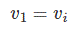Therefore, equation (i) can be rewritten as,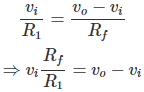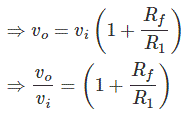The closed-loop gain of the circuit is,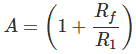This term does not contain any negative parts. Hence, it proves that the input signal to the circuit gets amplified without changing its polarity at the output.

From the expression of the voltage gain of a non-inverting op-amp, it is clear that the gain will be unity when Rf = 0 or R1 → ∝.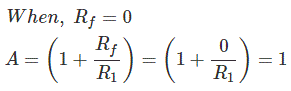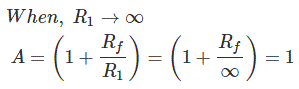So, if we short circuit the feedback path and/or open the external resistance of the inverting pin, the gain of the circuit becomes 1.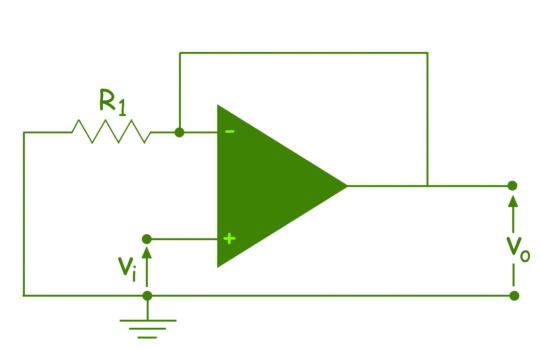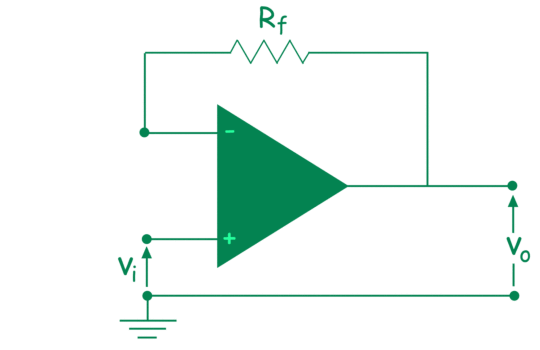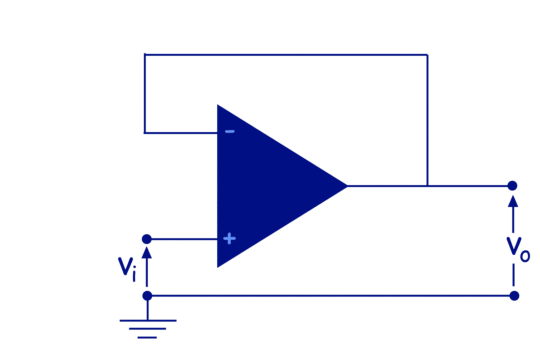This circuit is called a voltage follower or unity gain amplifier. This is used to isolate two cascaded circuits, because of its infinitely large impedance, at op-amp inputs.

Want To Learn Faster? 🎓
Get electrical articles delivered to your inbox every week.
No credit card required—it’s 100% free.